# How to use the INDEX function in Excel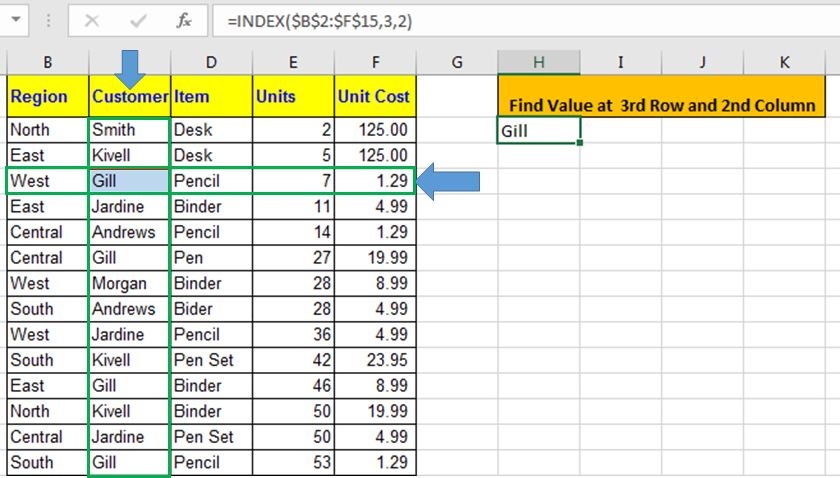In this article, we will learn How to use the INDEX function in Excel.

Why do we use the INDEX function ?

Given a table of 500 rows and 50 columns and we need to get a value at 455th row and 26th column. For this either we can scroll down to the 455th row and traverse to the 26th column and copy the value. But we can't treat Excel like hard copies. Index function returns the value at a given row and column index in a table array. Let's learn the INDEX function Syntax and illustrate how to use the function in Excel below.

INDEX Function in Excel

Index function returns the cell value at matching row and column index in array.

Syntax:

 =INDEX(array, row number, [optional column number])

array : It is the range or an array.

row number : Ther row number in your array from which you want to get your value.

column number : [optional] This column number in array. It is optional. If omitted INDEX formula automatically takes 1 as default.

Excel’s INDEX function has two forms known as:

• Array Form INDEX Function
• Reference Form INDEX Function

Example :

All of these might be confusing to understand. Let's understand how to use the function using an example. Here we have this data.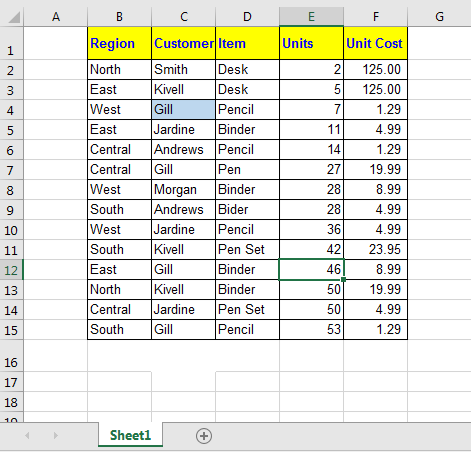I want to retrieve data at the intersection of 3rd Row and 2nd Column. I write this INDEX formula in cell H2:

 =INDEX(\$B\$2:\$F\$15,3,2)

The result is Gill: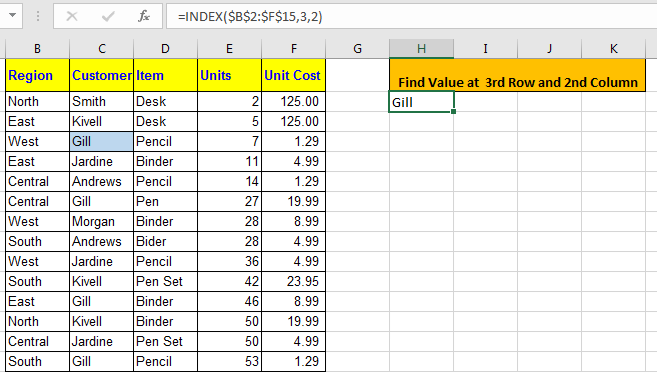Reference Form INDEX Function

It is much like a multidimensional array index function. Actually in this form of INDEX function, we can give multiple arrays and then in the end we can tell the index from which array to pull data.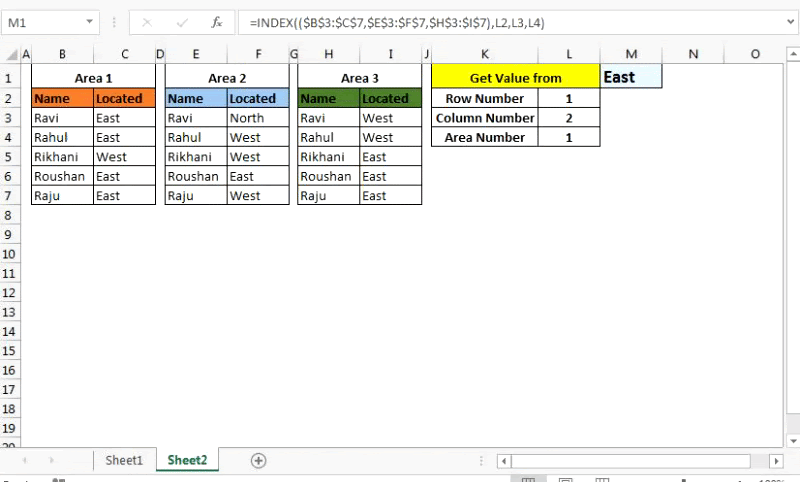Excel INDEX Function Reference Form Syntax

 =INDEX( (array1, array2,...), row number,  [optional column number],  [optional array number] )

(array1, array2,...) : This parenthesis contains a list of arrays. For example (A1:A10,D1:R100,...).

Row number : Ther row number in your array from which you want to get your value.

[optional column number] : This column number in array. It is optional. If omitted, the INDEX formula automatically takes 1 for it.

[optional array number] : The area number from which you want to pull data. In excel it is shown as area_num

Come, let’s have an example.

I have these 3 tables in Excel Worksheet.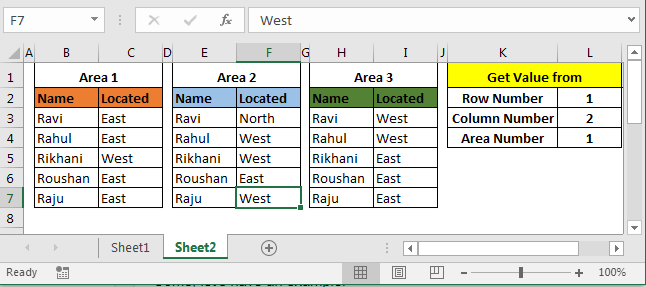Area 1, Area 2 and Area 3 are my ranges as shown in the above image. I need to retrieve data according to values in cell L2, L3 and L4. So, I write this INDEX formula in cell M1.

 =INDEX((\$B\$3:\$C\$7,\$E\$3:\$F\$7,\$H\$3:\$I\$7),L2,L3,L4)

Here L2 is 1, L3 is 2 and L4 is 1. Hence the INDEX function will return the value from the 1st row of the second column from the 1st array. And that is East.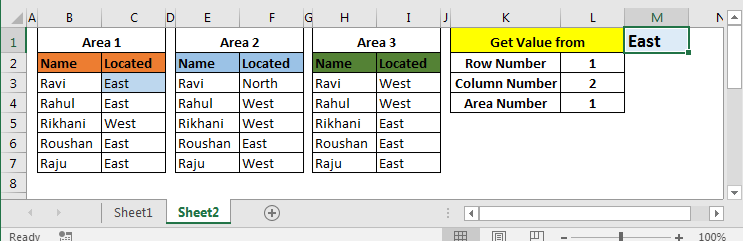Now change L2 to 2 and L4 to 2. You will have West in M2, as shown in below image.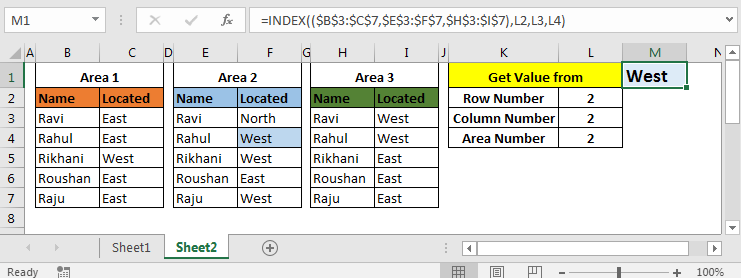And so on.

The INDEX function in Excel is mostly used with MATCH Function. The INDEX MATCH function is so famous that it is sometimes thought of as one single function. Here I have explained the INDEX MATCH function with multiple criteria in detail. Go check it out. How to lookup values using the INDEX and MATCH function

Hope this article about How to use the INDEX function in Excel is explanatory. Find more articles on calculating values and related Excel formulas here. If you liked our blogs, share it with your friends on Facebook. And also you can follow us on Twitter and Facebook. We would love to hear from you, do let us know how we can improve, complement or innovate our work and make it better for you. Write to us at info@exceltip.com.

Related Articles :

Use INDEX and MATCH to Lookup Value : The INDEX & MATCH formula is used to lookup dynamically and precisely a value in a given table. This is an alternative to the VLOOKUP function and it overcomes the shortcomings of the VLOOKUP function.

Use VLOOKUP from Two or More Lookup Tables : To lookup from multiple tables we can take an IFERROR approach. To lookup from multiple tables, it takes the error as a switch for the next table. Another method can be an If approach.

How to do Case Sensitive Lookup in Excel : The excel's VLOOKUP function isn’t case sensitive and it will return the first matched value from the list. INDEX-MATCH is no exception but it can be modified to make it case sensitive.

Lookup Frequently Appearing Text with Criteria in Excel : The lookup most frequently appears in text in a range we use the INDEX-MATCH with MODE function.

Popular Articles :

How to use the IF Function in Excel : The IF statement in Excel checks the condition and returns a specific value if the condition is TRUE or returns another specific value if FALSE.

How to use the VLOOKUP Function in Excel : This is one of the most used and popular functions of excel that is used to lookup value from different ranges and sheets.

How to use the SUMIF Function in Excel : This is another dashboard essential function. This helps you sum up values on specific conditions.

How to use the COUNTIF Function in Excel : Count values with conditions using this amazing function. You don't need to filter your data to count specific values. Countif function is essential to prepare your dashboard.

Terms and Conditions of use

The applications/code on this site are distributed as is and without warranties or liability. In no event shall the owner of the copyrights, or the authors of the applications/code be liable for any loss of profit, any problems or any damage resulting from the use or evaluation of the applications/code.